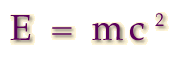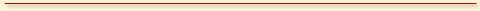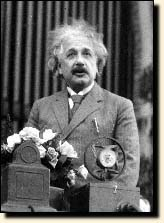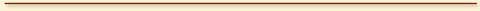## Einstein Explains the Equivalence of Energy and Matter### To listen to Albert Einstein explain his famous formula:"It followed from the special theory of relativity that mass and energy are both but different manifestations of the same thing -- a somewhat unfamiliar conception for the average mind. Furthermore, the equation E is equal to m c-squared, in which energy is put equal to mass, multiplied by the square of the velocity of light, showed that very small amounts of mass may be converted into a very large amount of energy and vice versa. The mass and energy were in fact equivalent, according to the formula mentioned above. This was demonstrated by Cockcroft and Walton in 1932, experimentally."From the soundtrack of the film, Atomic Physics.E=mc² Brought to you by The Center for History of Physics © Copyright 1996 - American Institute of Physics